# Building an easy Line Follower Robot using Arduino Uno

Published  September 8, 2021   2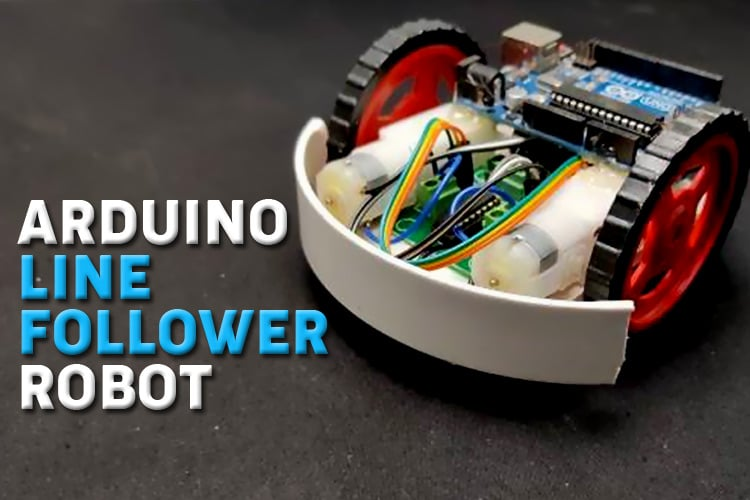Line Follower Robot (LFR) is a simple autonomously guided robot that follows a line drawn on the ground to either detect a dark line on a white surface or a white line on a dark. The LFR is quite an interesting project to work on! In this tutorial, we will learn how to build a black line follower robot using Arduino Uno and some easily accessible components. Sounds intersting? Let's get started. You can see the demonstration video of the Arduino Line Following robot-

### Working of a Line Follower Robot

As stated earlier, line follower robot (LFR) follows a line, and in order to follow a line, robot must detect the line first. Now the question is how to implement the line sensing mechanism in a LFR. We all know that the reflection of light on the white surface is maximum and minimum on the black surface because the black surface absorbs maximum amount of light. So, we are going to use this property of light to detect the line. To detect light, either LDR (light-dependent resistor) or an IR sensor can be used. For this project, we are going with the IR sensor because of its higher accuracy. To detect the line, we place two IR sensors one on the left and other on the right side of the robot as marked in the diagram below. We then place the robot on the line such that the line lies in the middle of both sensors. We have covered a detailed Arduino IR sensor tutorial which you can check to learn more about the working of IR sensors with Arduino Uno.

Infrared sensors consist of two elements, a transmitter and a receiver. The transmitter is basically an IR LED, which produces the signal and the IR receiver is a photodiode, which senses the signal produced by the transmitter. The IR sensors emits the infrared light on an object, the light hitting the black part gets absorbed thus giving a low output but the light hitting the white part reflects back to the transmitter which is then detected by the infrared receiver, thereby giving an analog output. Using the stated principle, we control the movement of the robot by driving the wheels attached to the motors, the motors are controlled by a microcontroller.

### How does a Line Follower Robot Navigates?

A typical line follower robot has two sets of motors, let's call them left motor and right motor. Both motors rotate  on the basis of the signal received from the left and the right sensors respectively. The robot needs to perform 4 sets of motion which includes moving forward, turning left, turning right and coming to a halt. The description about the cases are given below.

Moving Forward: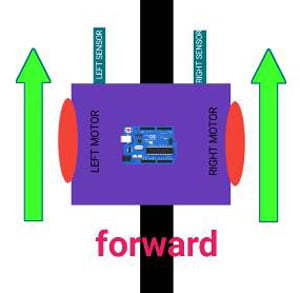In this case, when both the sensors are on a white surface and the line is between the two sensors, the robot should move forward, i.e., both the motors should rotate such that the robot moves in forward direction (actually both the motors should rotate in the opposite direction due to the placement of motors in our setup. But for the sake of simplicity, we will call the motors rotating forward.)

Turning LEFT: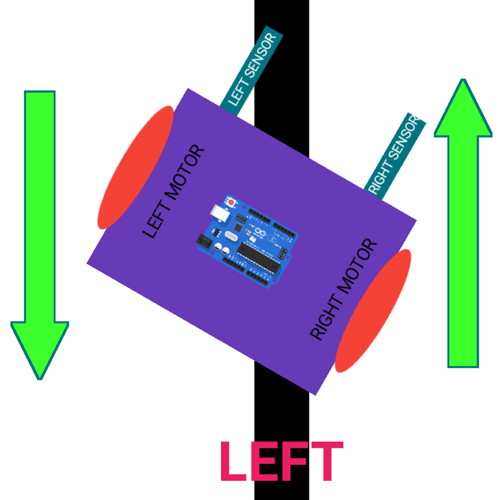In this case, the left sensor is on top of the dark line, whereas the right sensor is on the white part, hence the left sensor detects the black line and gives a signal, to the microcontroller. Since, signal comes from the left sensor, the robot should turn to the left direction. Therefore, the left motor rotates backwards and the right motor rotates in forward direction. Thus, the robot turns towards left side.

Turning RIGHT: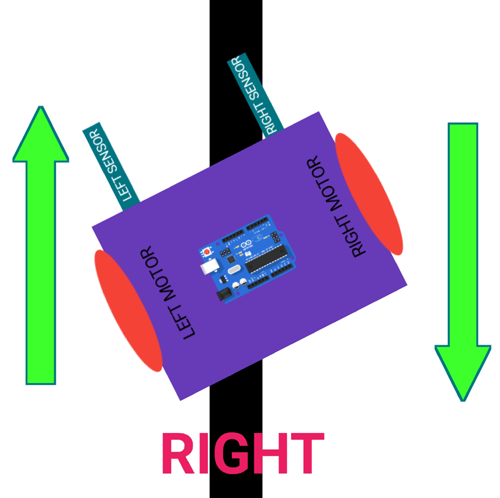This case is similar to the left case, but in this situation only the right sensor detects the line which means that the robot should turn in the right direction. To turn the robot towards the right direction, the left motor rotates forward and the right motor rotates backwards and as a result, the robot turns towards the right direction.

Stopping: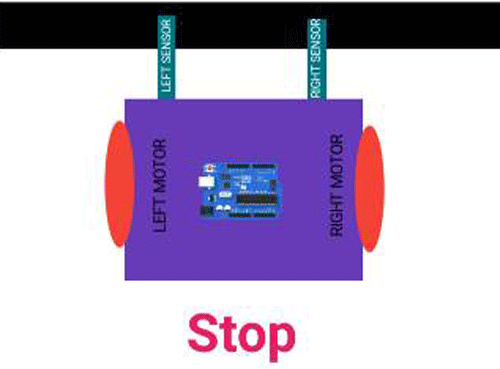In this case, both the sensors are on top of the line and they can detect the black line simultaneously, the microcontroller is fed to consider this situation as a process for halt. Hence, both the motors are stopped, which causes the robot to stop moving.

### Components Required for Making Arduino Line Follower Robot

1. Arduino Uno - 1Nos
2. L293D motor driver- 1Nos
3. IR sensor module -2 Nos
4. 7.4 or 9V battery -1 Nos
5. BO motor - 2 Nos
6. Motor wheel - 2 Nos
7. Castor wheel - 1 Nos
8. Hobby robot chassis - 1 Nos
9. Wires
10. Screw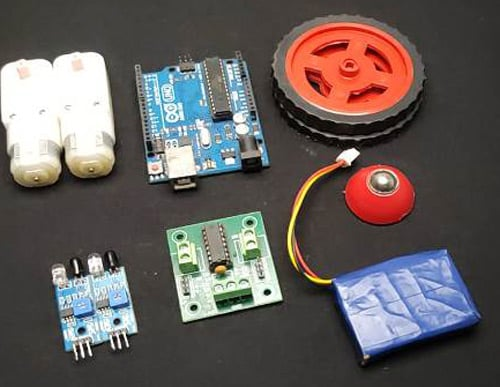### Circuit Diagram and Assembling the Arduino based Line Follower Robot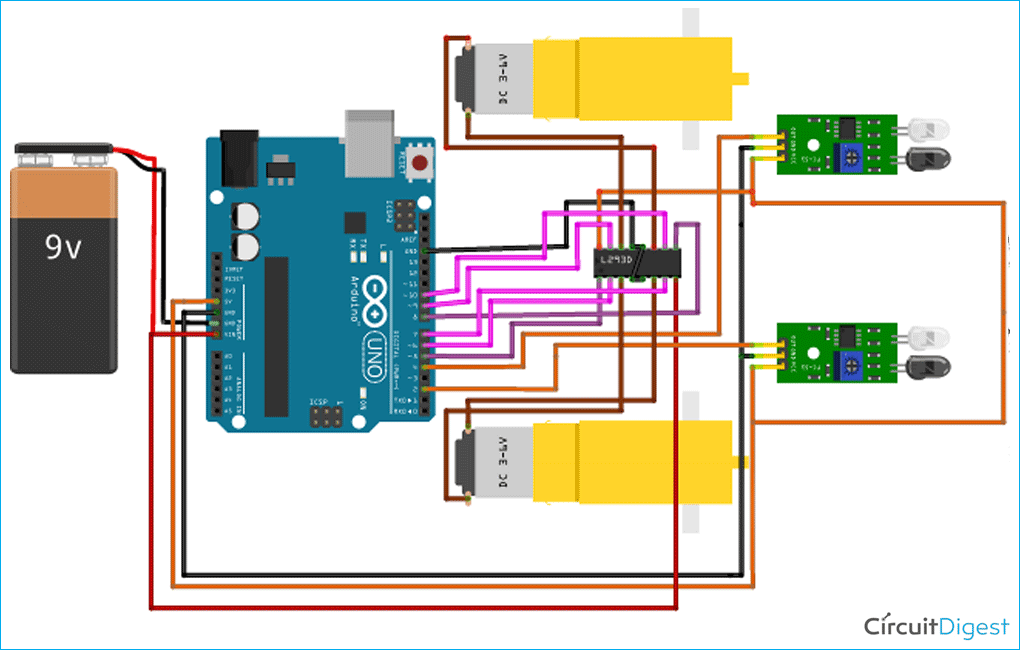The circuit consists of mainly four parts: Two IR sensors, one motor drive, two motors, one Arduino, a battery and few connecting wires. The sensor senses the IR light reflected from the surface and feeds the output to the onboard op-amp comparator. When the sensor is situated over the white background, the light emitted by the sensor is reflected by the white ground and is received by the receiver. But when the sensor is above the black background, the light from the source doesn’t reflect to it. The sensor senses the intensity of reflected light to give an output. The sensor’s output is fed to the microcontroller, which gives commands to the motor driver to drive the motor accordingly. In our project, the Arduino Uno is programmed to make the robot move forward, turn right or turn left and stop according to the input coming from the sensor. The output of the Arduino is fed to the motor driver.

Why We Require a Motor Driver?

The reason to use a motor driver here is because the output signal of an Arduino is not sufficient to drive the motor, furthermore, we need to rotate the motors in both directions, therefore we use a motor driver to drive the motor as required and also the motor driver is able to supply sufficient current to drive the motor. Here, we are using a L293D motor driver which is a dual h bridge motor driver and is sufficient for our 2 motors.

The L293D has 16 pins, the pinout of L293D is shown in the below diagram.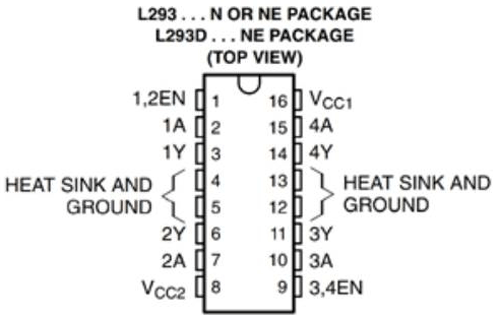Connection of motor driver pins are as follows:

Pin number 1 and 9 are the enable pins, we connect these two pins to a 5v input to enable the motor.

Pin number 1A, 2A, 3A, and 4A are the control pins.

For eg. The motor will turn to the right if the pin 1A goes low and 2A goes high, and the motor will turn to the left if 1A goes low and 2A high. So, we connect these pins to the output pins of the decoder.

Pins 1Y, 2Y, 3Y, and 4Y are the motor connection pins.

Note: Vcc2 is the motor driving voltage pin, and only used if you are using a high voltage motor.

Pin connection of Arduino Uno with the Motor driver are as follows: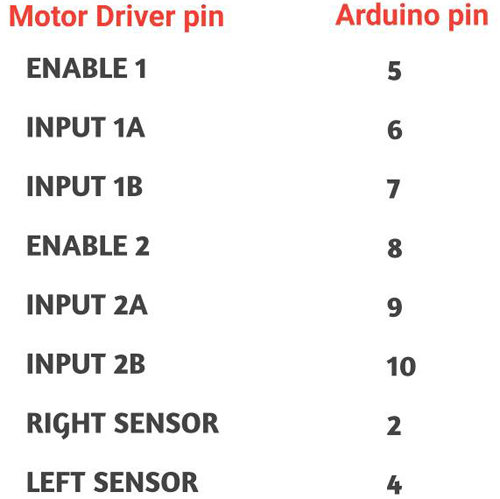Here, we are using a 7.4 li-ion battery to power the whole circuit. You can use any battery type from 6-12 volt. To move the robot, we need to use motors with low RPM but torque high enough to carry the weight of the robot. So, I chose two 60 RPM 6V Battery Operated, geared motors for this robot.

### Assembling the Line Follower Robot

Once we have understood the connection of all the components, we can start assembling our LFR. I have explained the step-by-step assembly procedure of the Robot in the video provided at the bottom of the page.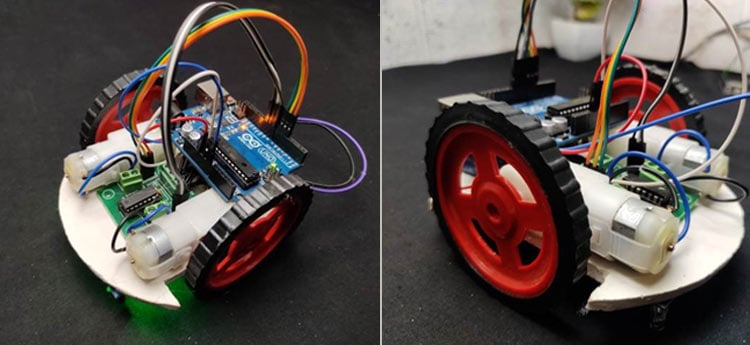To make this robot, first we need a robot body; here I am using a homemade chassis. You can either use a readymade chassis or build one yourself.

Now, place the BO motors to the chassis with the help of some hot glue as shown in the image below.Next step is to place the motor driver on chassis and connect the motor wires to the output of the motor driver.

Next, bend the IR LED and sensor as shown in the image.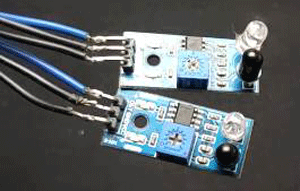Then place the sensors on the downside of the robot, adjust the sensors according to the track width and robot width. Remember that one sensor is for left side detection and another is for the right side detection.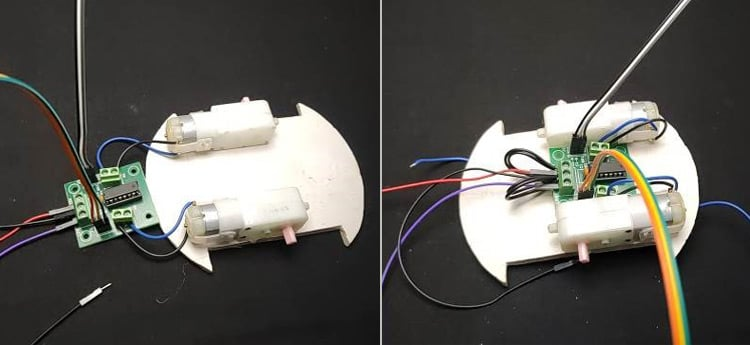Now place the Arduino uno using glue and connect the sensor output pins to digital pin 2 and 4 of the Arduino.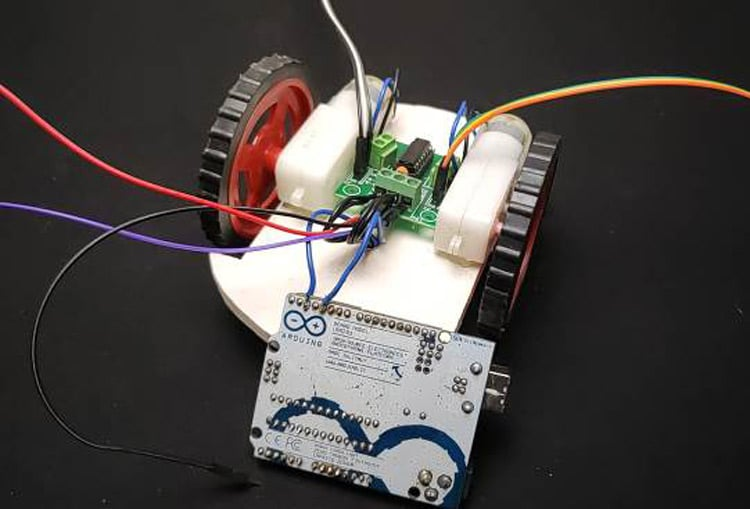Connect the VCC pins to 5volt and the ground pins to ground.

Now, connect the enable pins of the motor driver to pin 5 and 8 of Arduino and connect the motor driver input pins to pin number 6, 7, 9 and 10 of Arduino respectively.

Finally, connect the battery with the circuit and place the battery on chassis. Here, I have connected everything with jumper wires. To make a permanent setup, you can directly solder everything together.

Now turn the board upside down and with the help of hot glue gun, attach the castor wheels as shown in the image below.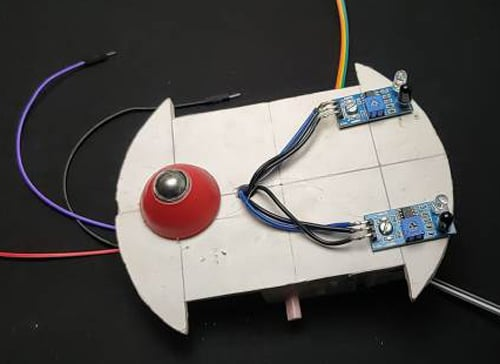Finally, add the wheels. For extra safety, I have added a plastic sheet as a bumper too.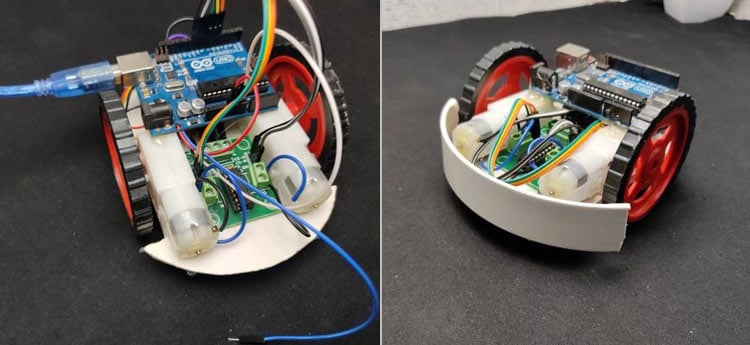### Arduino Line Follower Robot Code

The programming part of line follower robot is very simple and we require only basic Arduino functions. The complete program for this project can be found at the bottom of this page. The explanation of this program is as follows:

First step is to defined every Arduino pin that we are using. I started with motoring the driver pins and sensor pins. Here, I have commented on each line of code for your easy understanding.

``` #define enA 5 //Enable1 L293 Pin enA
#define in1 6 //Motor1  L293 Pin in1
#define in2 7 //Motor1  L293 Pin in1
#define in3 9 //Motor2  L293Pin in1
#define in4 10 //Motor2  L293 Pin in1
#define enB 8 //Enable2 L298 Pin enB
#define R_S 4 // Right sensor
#define L_S 2 // Left sensor```

In the loop section, declare the pin modes of each pin. Here, we need to read the output of IR sensors, hence I have defined those pins as an input. The motor needs to be driven by the Arduino, thus defining the motor driver pins as output. Finally, I pulled enable pin to high.

```pinMode(R_S, INPUT);
pinMode(L_S, INPUT);
pinMode(enA, OUTPUT);
pinMode(in1, OUTPUT);
pinMode(in2, OUTPUT);
pinMode(in3, OUTPUT);
pinMode(in4, OUTPUT);
pinMode(enB, OUTPUT);
digitalWrite(enA, HIGH);
digitalWrite(enB, HIGH);```

In the loop section, we first read the values of IR sensors and then using ‘if condition’ control the movement of motor as per our requirements. The four movement conditions are explained below.

Moving forward:

`if((digitalRead(R_S) == 0)&&(digitalRead(L_S) == 0)){forward();} `

In this case, if Right Sensor and Left Sensor are on top of white colour then the robot should move in the forward direction, so we call the forward function.

(Note : here 0 means output of IR sensor is high as the sensor is on white surface)

Turning towards Right Side:

`if((digitalRead(R_S) == 1)&&(digitalRead(L_S) == 0)){turnRight();} `

if Right Sensor is detecting Black and Left Sensor is not detecting any black line, then it will call the Right function, initiating the protocol for making right turn by calling turnRight function.

Turning towards Left side:

` if((digitalRead(R_S) == 0)&&(digitalRead(L_S) == 1)){turnLeft();} `

if Right Sensor is over white and the Left Sensor is detecting Black then it will call turnLeft function. It will initiate the steps for turning the robot in left direction.

Stopping the Robot:

` if((digitalRead(R_S) == 1)&&(digitalRead(L_S) == 1)){Stop();} `

If the Right Sensor and Left Sensor are on the Black color then it will call the Stop function. In this case, the robot will come to a complete halt.

We have defined the 4 functions of the robot as Forward, turnLeft, turnRight and Stop. The code to perform these functions are given below:

Forward function:

```void forward(){
digitalWrite(in1, HIGH);
digitalWrite(in2, LOW);
digitalWrite(in3, LOW);
digitalWrite(in4, HIGH);
}```

This means we are pulling to high the input 1 and 4 of the motor driver, as a result, both motors will move forward  same as this I made other functions

Turnright function:

```void turnRight(){
digitalWrite(in1, LOW);  //Right Motor forword Pin
digitalWrite(in2, HIGH); //Right Motor backword Pin
digitalWrite(in3, LOW);  //Left Motor backword Pin
digitalWrite(in4, HIGH); //Left Motor forword Pin
}```

TurnLeft function:

```void turnLeft(){
digitalWrite(in1, HIGH); //Right Motor forword Pin
digitalWrite(in2, LOW);  //Right Motor backword Pin
digitalWrite(in3, HIGH); //Left Motor backword Pin
digitalWrite(in4, LOW);  //Left Motor forword Pin
}```

Stop function:

```void Stop(){
digitalWrite(in1, LOW); //Right Motor forword Pin
digitalWrite(in2, LOW); //Right Motor backword Pin
digitalWrite(in3, LOW); //Left Motor backword Pin
digitalWrite(in4, LOW); //Left Motor forward Pin```

So, that’s all about coding. Now we need to upload the code to the Arduino and to do so, connect your Arduino with the computer using USB cable and upload this code using the Arduino IDE.

Testing and Calibration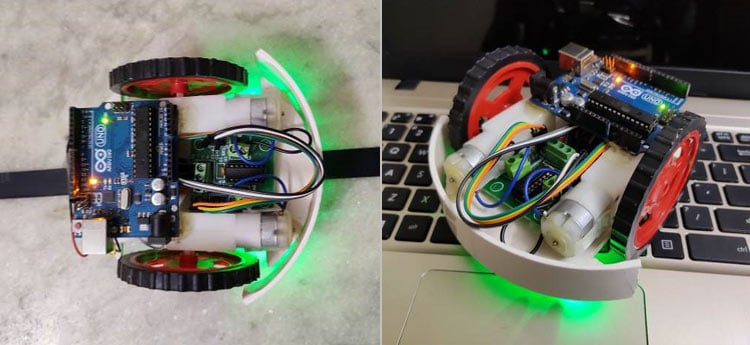We have assembled the robot and uploaded the code, so now its time to see it in action and if it is unable to follow the line then we’ll have to calibrate the robot. For that first place robot on a  black surface ( both sensors should be on top of the black surface) then adjust the variable resistor of IR Module until the output led of IR module become off. Next, place the robot on a white surface and check whether the led is turning on, if not, then just adjust the variable resistor. Repeat the process once again to be sure that the output LED is operating as per the requirement.

Now, since we have calibrated the robot, all we need to do is place the robot on top of the black line and see it in action.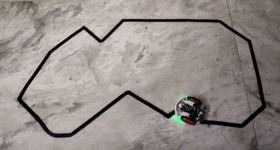The complete making of the line follower robot can be found in the video linked at the bottom of this page. If you have any questions leave them in the comment section.

Code

// Arduino Line Follower Robot Code

#define enA 5//Enable1 L293 Pin enA
#define in1 6 //Motor1  L293 Pin in1
#define in2 7 //Motor1  L293 Pin in1
#define in3 9 //Motor2  L293 Pin in1
#define in4 10 //Motor2  L293 Pin in1
#define enB 8 //Enable2 L293 Pin enB
#define R_S 4//ir sensor Right
#define L_S 2 //ir sensor Left
void setup(){
pinMode(R_S, INPUT);
pinMode(L_S, INPUT);
pinMode(enA, OUTPUT);
pinMode(in1, OUTPUT);
pinMode(in2, OUTPUT);
pinMode(in3, OUTPUT);
pinMode(in4, OUTPUT);
pinMode(enB, OUTPUT);
digitalWrite(enA, HIGH);
digitalWrite(enB, HIGH);
delay(1000);
}
void loop(){
if((digitalRead(R_S) == 0)&&(digitalRead(L_S) == 0)){forward();}   //if Right Sensor and Left Sensor are at White color then it will call forword function
if((digitalRead(R_S) == 1)&&(digitalRead(L_S) == 0)){turnRight();} //if Right Sensor is Black and Left Sensor is White then it will call turn Right function
if((digitalRead(R_S) == 0)&&(digitalRead(L_S) == 1)){turnLeft();}  //if Right Sensor is White and Left Sensor is Black then it will call turn Left function
if((digitalRead(R_S) == 1)&&(digitalRead(L_S) == 1)){Stop();} //if Right Sensor and Left Sensor are at Black color then it will call Stop function
}
void forward(){  //forword
digitalWrite(in1, HIGH); //Right Motor forword Pin
digitalWrite(in2, LOW);  //Right Motor backword Pin
digitalWrite(in3, LOW);  //Left Motor backword Pin
digitalWrite(in4, HIGH); //Left Motor forword Pin
}
void turnRight(){ //turnRight
digitalWrite(in1, LOW);  //Right Motor forword Pin
digitalWrite(in2, HIGH); //Right Motor backword Pin
digitalWrite(in3, LOW);  //Left Motor backword Pin
digitalWrite(in4, HIGH); //Left Motor forword Pin
}
void turnLeft(){ //turnLeft
digitalWrite(in1, HIGH); //Right Motor forword Pin
digitalWrite(in2, LOW);  //Right Motor backword Pin
digitalWrite(in3, HIGH); //Left Motor backword Pin
digitalWrite(in4, LOW);  //Left Motor forword Pin
}
void Stop(){ //stop
digitalWrite(in1, LOW); //Right Motor forword Pin
digitalWrite(in2, LOW); //Right Motor backword Pin
digitalWrite(in3, LOW); //Left Motor backword Pin
digitalWrite(in4, LOW); //Left Motor forword Pin
}

Video

Tags